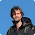## Tuesday, September 4, 2012

### Phylogenetic signal on very small trees

A phytools user recently contacted me about estimating phylogenetic signal for data collected for the taxa in a very small (n=7, in this case) phylogenetic tree.

In general this will not be a very good idea. With only seven or a similarly small number of tips there is simply not enough information about the correlations between related species, which is what we use to estimate the phylogenetic signal in our dataset.

This cannot be solved by devising a "better" method for computing signal. Attempting to do so would be somewhat akin to looking for a better way to estimate the population mean from a sample (than, say, the arithmetic mean) when our estimate has high variance entirely due to the smallness of our sample size.

One way to illustrate this general problem would be to simulate under the null hypothesis (or under some alternative hypothesis for signal), and then estimate signal. Power to estimate phylogenetic signal will depend on the structure of our tree, of course, so let's average over this uncertainty by simulating (say) 1000 independent trees & datasets, and then estimating signal for each case.

First, with Pagel's λ:

> library(phytools)
> # now simulate 1000 trees
> N<-7
> trees<-pbtree(n=N,nsim=1000)
> # simulate data
> X<-lapply(trees,fastBM)
> # find MLE(lambda) for each tree
> lambda<-mapply(function(x,y)
phylosig(x,y,method="lambda")\$lambda,x=trees,y=X)
> # compute mean lambda & plot
> h<-hist(lambda,-0.5:ceiling(max(lambda)*10.5)/10,
main=paste("N =",N))
> lines(c(mean(lambda),mean(lambda)),c(0,1.1*max(h\$counts)), lty="dashed")

We can see that our MLEs of λ are all over the place. Estimation is bounded by 0 and the maximum value of λ for which the likelihood function is defined. We also have a huge mode (because of our bounds) near λ = 0. Wow. Now let's compare to estimation on a very modest sized (say, n = 50) phylogeny:

> N<-50
> trees<-pbtree(n=N,nsim=1000)
> X<-lapply(trees,fastBM)
> lambda<-mapply(function(x,y) phylosig(x,y,method="lambda")\$lambda,x=trees,y=X)
> h<-hist(lambda,-0.5:ceiling(max(lambda)*20.5)/20,
main=paste("N =",N))
> lines(c(mean(lambda),mean(lambda)),c(0,1.1*max(h\$counts)), lty="dashed")

So, the picture is already quite different for even a smallish (but not tiny) tree.

We can also simulate under an intermediate level of phylogenetic signal and conduct the same test. Let's say, λ = 0.6:

> library(geiger)
> N<-7; l<-0.6
> trees<-pbtree(n=N,nsim=1000)
> t<-lapply(trees,lambdaTree,lambda=l)
> X<-lapply(t,fastBM)
> lambda<-mapply(function(x,y) phylosig(x,y,method="lambda")\$lambda,x=trees,y=X)
> h<-hist(lambda,-0.5:ceiling(max(lambda)*10.5)/10,
main=paste("N =",N,", lambda =",l))
> lines(c(mean(lambda),mean(lambda)),c(0,1.1*max(h\$counts)), lty="dashed")

Yikes. Let's try it, once again, for our modest sized trees:

> N<-50; l<-0.6
> trees<-pbtree(n=N,nsim=1000)
> t<-lapply(trees,lambdaTree,lambda=l)
> X<-lapply(t,fastBM)
> lambda<-mapply(function(x,y) phylosig(x,y,method="lambda")\$lambda,x=trees,y=X)
> h<-hist(lambda,-0.5:ceiling(max(lambda)*20.5)/20,
main=paste("N =",N,", lambda =",l))
> lines(c(mean(lambda),mean(lambda)),c(0,1.1*max(h\$counts)), lty="dashed")

Now (at least!) the distribution is more or less centered on the generating value of λ, in this case 0.6.

We can do the same kind of thing with Blomberg et al.'s (2003) K. Here, though, it is not obvious how to simulate under a particular value of K, so let's just simulate Brownian evolution [E(K)=1.0 under BM] for various N.

> N<-7
> trees<-pbtree(n=N,nsim=1000)
> X<-lapply(trees,fastBM)
> K<-mapply(phylosig,trees,X)
> h<-hist(K,-0.5:(5*10.5)/10,main=paste("K, N =",N))
> lines(c(mean(K),mean(K)),c(0,1.1*max(h\$counts)),lty="dashed")

Again, for small sample sizes we would expect a wide range of estimated phylogenetic signal, although in this case K is unbiased across the range of N simulated.

That's it!

1.2.